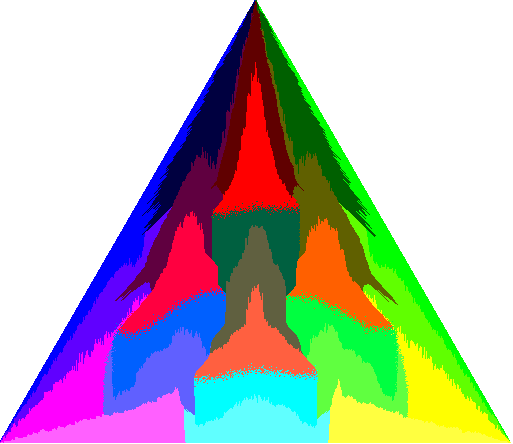That Geometry ShowLesson 1: What is Geometry? Points, Lines, & Planes (Notes, Key)Lesson 2: Segment Measurement, Midpoints, & Congruence (Notes, Key)Lesson 3: Angles - Exploration & Relationships (Notes, Key)Lesson 4: If-Then Statements & Deductive Reasoning (Notes, Key)Lesson 5: Parallel Lines, Transversals, & Angles (Notes, Key)Lesson 6: Slope (Notes, Key)Lesson 7: Distance Between Parallel Lines (Notes, Key)Lesson 8: Classifying Triangles & Angle Measure (Notes, Key)Lesson 9: Exploring Congruent Triangles (Notes, Key)Lesson 10: Analyzing Isosceles Triangles (Notes, Key)Lesson 11: Special Triangle Segments (Notes, Key)Lesson 12: Right Triangles & The Pythagorean Theorem (Notes, Key)Lesson 13: The Triangle Inequality (Notes, Key)Lesson 14: Parallelograms & Tesselations (Notes, Key)Lesson 15: Standardized Test Questions (Notes, Key)Lesson 16: Rectangles, Squares, & Rhombi (Notes, Key)Lesson 17: Trapezoids (Notes, Key)Lesson 18: Similarity & Proportionality (Notes, Key)Lesson 19: Similar Triangles (Notes, Key)Lesson 20: Parallel Lines & Proportional Parts (Notes, Key)Lesson 21: Polygons & Area (Notes, Key)Lesson 22: 3-D Figures & Polyhedra (Notes, Key)Lesson 23: Nets & Surface Area (Notes, Key)Lesson 24: Surface Area - Prisms, Cylinders, Pyramids, & Cones (Notes, Key)Lesson 25: Volume - Prisms, Cylinders, Pyramids, & Cones (Notes, Key)Lesson 26: Spheres - Surface Area & Volume (Notes, Key)Lesson 27: Circles, Angles, & Arcs (Notes, Key)Lesson 28: Circles - Arcs, Chords, Inscribed Triangles, Area (Notes, Key)Lesson 29: Special Right Triangles (Notes, Key)Lesson 30: Trigonometric Ratios of Right Triangles (Notes, Key)Lesson 31: Other Types of Geometries (Notes)